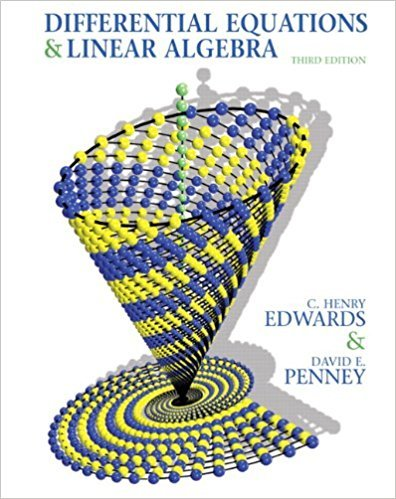×
×

# Solutions for Chapter 2.2: Differential Equations and Linear Algebra 3rd Edition## Full solutions for Differential Equations and Linear Algebra | 3rd Edition

ISBN: 9780136054252Solutions for Chapter 2.2

Solutions for Chapter 2.2
4 5 0 397 Reviews
17
1
##### ISBN: 9780136054252

This textbook survival guide was created for the textbook: Differential Equations and Linear Algebra, edition: 3. Chapter 2.2 includes 29 full step-by-step solutions. Since 29 problems in chapter 2.2 have been answered, more than 12660 students have viewed full step-by-step solutions from this chapter. Differential Equations and Linear Algebra was written by and is associated to the ISBN: 9780136054252. This expansive textbook survival guide covers the following chapters and their solutions.

Key Math Terms and definitions covered in this textbook
• Affine transformation

Tv = Av + Vo = linear transformation plus shift.

• Basis for V.

Independent vectors VI, ... , v d whose linear combinations give each vector in V as v = CIVI + ... + CdVd. V has many bases, each basis gives unique c's. A vector space has many bases!

• Big formula for n by n determinants.

Det(A) is a sum of n! terms. For each term: Multiply one entry from each row and column of A: rows in order 1, ... , nand column order given by a permutation P. Each of the n! P 's has a + or - sign.

• Cayley-Hamilton Theorem.

peA) = det(A - AI) has peA) = zero matrix.

• Cholesky factorization

A = CTC = (L.J]))(L.J]))T for positive definite A.

• Condition number

cond(A) = c(A) = IIAIlIIA-III = amaxlamin. In Ax = b, the relative change Ilox III Ilx II is less than cond(A) times the relative change Ilob III lib II· Condition numbers measure the sensitivity of the output to change in the input.

• Elimination.

A sequence of row operations that reduces A to an upper triangular U or to the reduced form R = rref(A). Then A = LU with multipliers eO in L, or P A = L U with row exchanges in P, or E A = R with an invertible E.

• Gram-Schmidt orthogonalization A = QR.

Independent columns in A, orthonormal columns in Q. Each column q j of Q is a combination of the first j columns of A (and conversely, so R is upper triangular). Convention: diag(R) > o.

• Hankel matrix H.

Constant along each antidiagonal; hij depends on i + j.

• Kronecker product (tensor product) A ® B.

Blocks aij B, eigenvalues Ap(A)Aq(B).

• Linear transformation T.

Each vector V in the input space transforms to T (v) in the output space, and linearity requires T(cv + dw) = c T(v) + d T(w). Examples: Matrix multiplication A v, differentiation and integration in function space.

• Pascal matrix

Ps = pascal(n) = the symmetric matrix with binomial entries (i1~;2). Ps = PL Pu all contain Pascal's triangle with det = 1 (see Pascal in the index).

• Projection matrix P onto subspace S.

Projection p = P b is the closest point to b in S, error e = b - Pb is perpendicularto S. p 2 = P = pT, eigenvalues are 1 or 0, eigenvectors are in S or S...L. If columns of A = basis for S then P = A (AT A) -1 AT.

• Projection p = a(aTblaTa) onto the line through a.

P = aaT laTa has rank l.

• Rank r (A)

= number of pivots = dimension of column space = dimension of row space.

• Reflection matrix (Householder) Q = I -2uuT.

Unit vector u is reflected to Qu = -u. All x intheplanemirroruTx = o have Qx = x. Notice QT = Q-1 = Q.

• Rotation matrix

R = [~ CS ] rotates the plane by () and R- 1 = RT rotates back by -(). Eigenvalues are eiO and e-iO , eigenvectors are (1, ±i). c, s = cos (), sin ().

• Row space C (AT) = all combinations of rows of A.

Column vectors by convention.

• Solvable system Ax = b.

The right side b is in the column space of A.

• Symmetric factorizations A = LDLT and A = QAQT.

Signs in A = signs in D.

×